﻿ Microstructure Predictions
Manufacturing Solutions

# Microstructure Predictions# Microstructure PredictionsOne of the key benefits of HX-Forge solver is the ability to predict Microstructure. During hot deformation process, the billet may undergo recrystallization through any of the recrystallization mechanisms, namely, static, dynamic and meta-dynamic recrystallization. Dynamic recrystallization usually occurs during deformation where as static or meta-dynamic recrystallization takes place post deformation. In HX-forge solver, grain size is calculated as a post processing step using one of the above mentioned recrystallization mechanisms. Most commonly used Avrami type semi-empirical models that express grain size as functions of initial grain size, strain, strain rate and temperature are used in microstructure computations. These models are described below.

### Dynamic Recrystallization Model:

The grain size during dynamic recrystallization is calculated using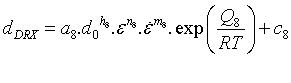Dynamically recrystallized fraction is given by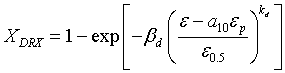Where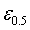is strain for 50% recrystallization and is given by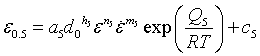And peak strain corresponds to maximum flow stress is given by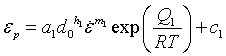The average grain size after dynamic recrystallization is calculated using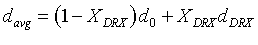### Static Recrystallization Model:

The grain size during static recrystallization is calculated using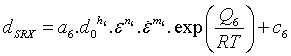Statically recrystallized fraction is given by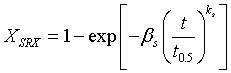where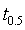is time constant for 50% recrystallization and is given by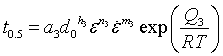The average grain size after static recrystallization is calculated using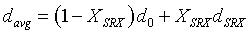### Meta-dynamic Recrystallization Model:

The grain size during meta-dynamic recrystallization is calculated using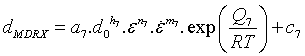Meta-dynamically recrystallized fraction is given by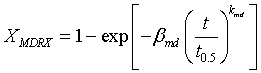whereis time constant for 50% recrystallization and is given by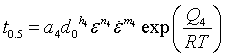The average grain size after meta-dynamic recrystallization is calculated using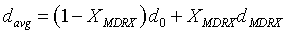In the above expressions,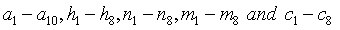are material coefficients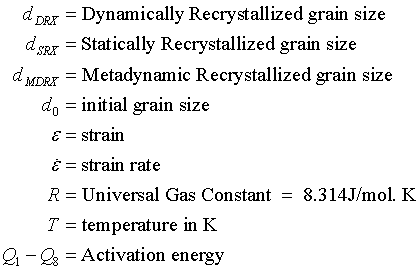### Yield Strength Calculation:

In HX-Solver, yield strength is calculated using Hall-Petch equation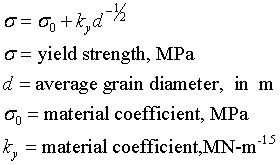In HX-Forge, although GUI to specify the data relevant to microstructure is not available at present (planned for future release), user can specify this data by manually editing the *.grf file. The data format required by HX-Forge solver is described below.

BEGIN PROBLEMDATA

MicrostructureData GSData  {

# Initial Grainsize in microns

InitialGrainSize         = 250

# Universal Gas Constant R in J/mol.K

UniversalGasConstant   = 8.314

MDRXModel           = "MDRX_Data"

YieldStrengthModelType  = "Hall_Petch"

YieldStrengthModel      = "Yield_Data"

}

MDRXGrainSizeData MDRX_Data {

A         = 0.0001

a4        = 5.043E-09

a7        = 4.85E+10

b1        = 250

b2        = 1

c7        = 0.0

h4        = 0.0

h7        = 0.0

n4        = -1.42

n7        = -0.41

m4        = -0.408

m7        = -0.028

Q2        = 0

Q4        = 196000

Q7        = -240000

beta_mdrx = 0.693

k_mdrx    = 1.0

}

YieldStrengthData Yield_Data {

sigma0  = 25.0

ky    = 0.5

# sigma0 is in   MPa

# ky     is in   MN m^-3/2

}

END

The different input parameters in the above data packet for microstructure predictions are explained below:

MicrostructureData is a keyword used to represent the data that include details about the recrystallization model, yield strength model and the associated material coefficients.

InitialGrainSize:

Initial grain size should be prescribed in microns.

UniversalGasConstant:

Always  8.314 J/mol. K

GrainSizeModelType:

For this parameter, user specifies a character string correspond to one of the recrystallization models described above as the model for grain size calculations.

The allowed values and corresponding recrystallization models are given below:

GrainSizeModelType  = “StaticRX”        – Static Recrystallization Model

GrainSizeModelType  = “DynamicRX”      – Dynamic Recrystallization Model used

GrainSizeModelType  = “MetaDynamicRX”  – Meta-dynamic  Recrystallization Model

Once the GrainSizeModelType is set, user should specify the material coefficients data for the respective recrystallization model. In the above example, GrainSizeModelType is set as “MetadynamicRX”, hence the solver expects the user to specify MDRXModel and the material coefficients data required for this model MDRXGrainSizeData as a separate data packet.

Meta-dynamic  Recrystallization data example:

MDRXModel       = "MDRX_Data"

MDRXGrainSizeData MDRX_Data {

A         = 0.0001

a4        = 5.043E-09

a7        = 4.85E+10

b1        = 250

b2        = 1

c7        = 0.0

h4        = 0.0

h7        = 0.0

n4        = -1.42

n7        = -0.41

m4        = -0.408

m7        = -0.028

Q2        = 0

Q4        = 196000

Q7        = -240000

beta_mdrx = 0.693

k_mdrx    = 1.0

}

Static Recrystallization data example:

GrainSizeModelType  = “StaticRX”

SRXModel       = "SRX_Data"

SRXGrainSizeData SRX_Data {

A       = 1000

a3       = 3.16

a6       = 678.0

b1       = 250

b2       = 1

c6       = 0.0

h3       = 0.0

h6       = 0.0

n3       = -0.75

n6       = 0.0

m3       = 0.0

m6       = 0.0

Q2       = 0

Q3       = 74826

Q6       = -31710

beta_srx = 0.0

k_srx    = 1.0

}

Dynamic Recrystallization data example:

GrainSizeModelType  = “DynamicRX”

DRXModel       = "DRX_Data"

DRXGrainSizeData DRX_Data {

a1       = 0

a2       = 0.0

a5       = 0.0

a8       = 8.26E09

c1       = 0.0

c5       = 0.0

c8       = 0.0

h1       = 0.0

h5       = 0.0

h8       =1.0

n1       = 0.0

n5       = 0.0

n8       = -0.420

m1       = 0.0

m5       =0.0

m8       = 0.046

Q1       = 0

Q5       =0.0

Q8       = 0.0

beta_drx = 0.0

k_drx    = 0.0

}

Yield Strength data example:

YieldStrengthData Yield_Data {

sigma0  = 25.0

ky      = 0.5

# sigma0 is in   MPa

# ky     is in   MN m^-3/2